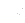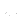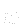Previous: Dynamic Mesh Up: Simulation and Evaluation set Next: Running the simulation

This is an automatically generated documentation by LaTeX2HTML utility. In case of any issue, please, contact us at info@cfdsupport.com.

## Evaluation of the torque

• The aim of this section is to set up an evaluation of the torque a possibly also the power. The torque evaluation shall run during the simulation and it shall write the actual value of the torque in each time-step into the output file.
• The power can be evaluated from the torque simply with just using following formula: \$P=&tau#tau;·&omega#omega;\$, whereis a power,is a torque andis an angular velocity of rotation.
• The so called function object – forces will be used for the torque evaluation. It calculates the forces and moments by integrating the pressure and the skin-friction forces over a given list of patches (usually wall boundaries).
• To enable the forces function object – one shall do the forces function object setup in the system/controlDict in section functions.
• There are two options how to do the function object setup.
1. The function object setup could be done directly – one shall just write the definition to the system/controlDict file.
2. The function object setup could be in independent text-file – one shall create a new text-file e.g. system/forces where one states the function object setup and then one includes the file forces into the controlDict.
• Let’s choose the second option due to easier readability and create a new file system/forces which shall contain following:
• Enumerate all the patches on which the torque shall be evaluated in the patches section (lines 16 – 32).
• Define the names of the variables which represents pressure, velocity and density (lines 33 – 36) – please note the density is defined as constant in this case because the simulation is incompressible.
• Origin of the axis of rotation is also necessary to define correctly (line 37).
• It is necessary to use a different coordinate system in this case, where the axis of rotation is not aligned with any of the principal axis. In the coordinateRotation section set coordinates of two base vectors of the coordinate system aligned with the axis of rotation – they are – e1 and e2 (lines 38-43).
• And finally let’s add the new file forces into the system/controlDict. – the listing of corresponding part of system/controlDict follows.
```functions
{
#include "forces"
}
```